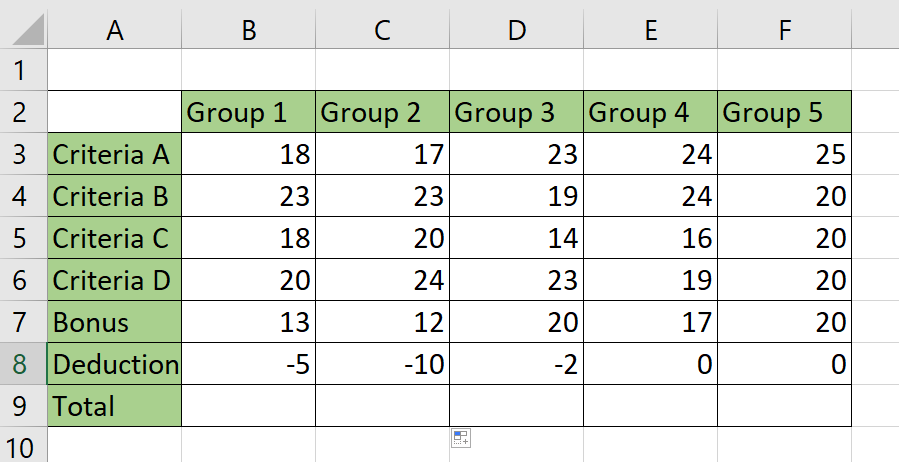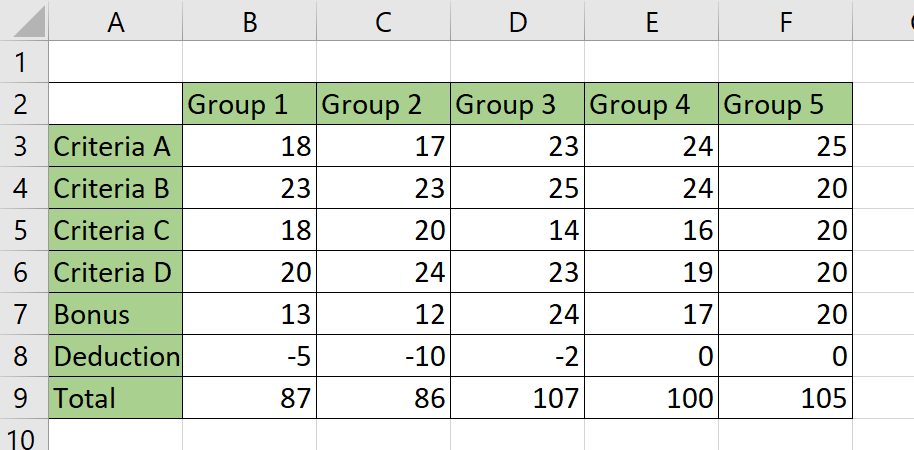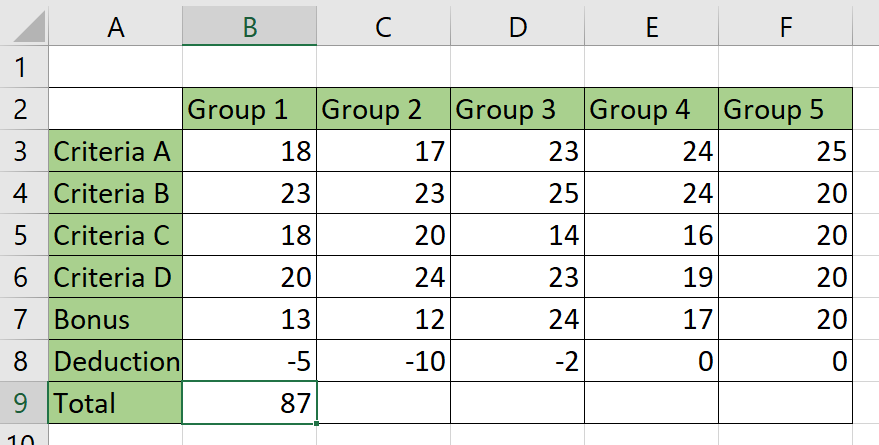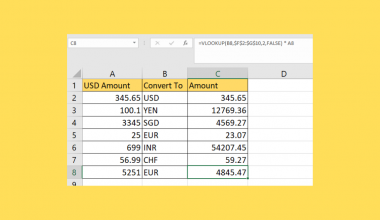# How to Prevent Your Formula from Exceeding a Certain Value in Excel

This guide will explain how to prevent your formula from exceeding a certain value in Excel.

We can use Excel functions to set an upper limit on the output of an Excel formula.

If users want to ensure that formula does not exceed a certain value, they can use the `MIN` function. The `MIN` function will take in a list of values and return the smallest value in that list.

We can place our target formula as our first argument and our limit as the second argument. The `MIN` formula will always choose whichever argument is lower.

Let’s take a look at a simple example where we can use the `MIN` function.

Suppose you are grading a classroom activity based on multiple criteria. The scoring system is set up in a way that it is possible for students to go over a score percentage of 100%.

The `MIN` function can help the user handle cases where the score exceeds 100%. This can be helpful since the maximum score for any classroom activity is 100%

Now that we know when to use the `MIN` function, let’s see how this works on an actual sample spreadsheet.

## A Real Example of Preventing Your Formula from Exceeding a Certain Value in Excel

The following section provides several examples of how to use the “How to Avoid Your Formula from Exceeding a Certain Value in Excel” function. We will also go into detail about the formulas and tools used in these examples.

First, let’s take a look at a real example of the function being used in an Excel spreadsheet.

You are in charge of computing the final score for a group activity in a university class. Each group is scored in four separate criteria from 1 to 25. In addition to these criteria, you also can grant any group bonus points or apply deductions for late submissions.If we try to use the `SUM` formula by itself, it’s possible to get scores over 100. How can we modify the formula so that the maximum score will always be 100?We can use the `MIN` function to add a limit to our score. If the sum of all the criteria, bonus points, and deductions is over 100, the `MIN` function will choose the value of 100 instead since it has a lower value.To get the values in the total row, we can use the following formula:

`=MIN(SUM(B3:B8);100)`

The `MIN` function includes two arguments: the sum of all the criteria, and the value 100. The function returns whichever argument is lower.

Do you want to take a closer look at our examples? You can make your own copy of the spreadsheet above using the link attached below.

If you’re ready to try out the `MIN` function to set an upper limit to a formula’s output, head over to the next section to read our step-by-step breakdown on how to do it!

## How to Prevent Your Formula from Exceeding a Certain Value in Excel

This section will guide you through each step needed to start using the `MIN` function to prevent formulas from exceeding a specified value.

Follow these steps to start using the `MIN` function:

1. First, select the cell you want to place your formula. In this example, we want to compute the total in cell B9.2. We’ll start with the `MIN` function before writing the actual formula that will perform the summation. Type ‘=MIN(‘ to start the `MIN` formula.3. Add the formula you want to limit as the first argument. In this example, we want to limit the result of SUM(B3:B8).4. Next, enter the value you want to use as the limit to the formula you’ve entered previously. In this example, we want our `SUM` function to have a maximum output of 100.5. Type the Enter key to evaluate the function.6. Use the Fill Handle tool to apply the formula to the rest of the row. Notice how Group 3 and Group 5 no longer have a score over 100.These are all the steps you need to prevent your formula from exceeding a certain value in Excel.

This step-by-step guide should provide you with all the information you need to begin using the `MIN` function to limit the output of another formula.

This technique is useful if you are computing a metric with a maximum possible value. For example, test scores are often capped at the value of 100.

Preventing your formula from exceeding a certain value is just one example of the many Excel features you can use in your spreadsheets.

Our website offers hundreds of other functions and methods to help you get more out of Microsoft Excel. With so many other Excel functions available, you can find one appropriate for your use case.

Don’t miss out on our team’s new spreadsheet tips, tricks, and best practices. Subscribe to our newsletter to stay updated on the latest guides from us!### Get emails from us about Excel.

Our goal this year is to create lots of rich, bite-sized tutorials for Excel users like you. If you liked this one, you'd love what we are working on! Readers receive ✨ early access ✨ to new content.

##### You May Also Like## How to Convert Time into Decimals in Excel

This guide will explain how to convert time into decimals in Excel using two simple methods. The rules…## How to Calculate an Exponential Moving Average in Excel

This guide will explain how to calculate an exponential moving average in Excel. Since it has several built-in…## How to Hide All Error Values in Excel

We can use the IFERROR function and conditional formatting to hide all error values in a Microsoft Excel…## How to Keep Leading Zeros in Excel CSV

This guide will explain how to keep leading zeros in Excel CSV using two simple methods. CSV or…